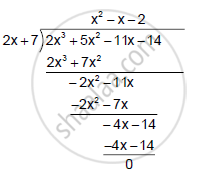Share

Using the Factor Theorem, Show That: (Iv) 2x + 7 is a Factor 2x3 + 5x2 − 11x – 14. Hence, Factorise the Given Expression Completely. - ICSE Class 10 - Mathematics

Question

Using the factor Theorem, show that:

(iv) 2x + 7 is a factor 2x3 + 5x2 − 11x – 14. Hence, factorise the given expression completely.

Solution

f(x) = 2x3 + 5x2 - 11x - 14
2x + 7 = 0  ⇒  x = ((-7)/2)

Remainder = f  ((-7)/2)

= 2  ((-7)/2)+ 5  ((-7)/2)^2 - 11  ((-7)/2) - 14

= (-343)/4 + 245/4 + 77/2 - 14

= (-49)/2 + 77/2 -14

= 28/2 -14

= 14 - 14=0

Hence, (2x + 7) is a factor of f(x).
Now, we have:∴ 2 x  ^ 3 +  5 x^2 - 11x -14 = (2x + 7 ) (x^2- x-2)

= (2x +  7)(x^2 - 2x + x -2 )

= (2x + 7 )[x(x-2)+(x-2)]

= (x + 7)(x-2)(x+1)

Is there an error in this question or solution?

Video TutorialsVIEW ALL 

Solution Using the Factor Theorem, Show That: (Iv) 2x + 7 is a Factor 2x3 + 5x2 − 11x – 14. Hence, Factorise the Given Expression Completely. Concept: Factor Theorem.
S